# Parcels Tutorial¶

Welcome to a quick tutorial on Parcels. This is meant to get you started with the code, and give you a flavour of some of the key features of Parcels.

In this tutorial, we will first cover how to run a set of particles from a very simple idealised field. We will show how easy it is to run particles in time-backward mode. Then, we will show how to add custom behaviour to the particles. Then we will show how to run particles in a set of NetCDF files from external data. Then we will show how to use particles to sample a field such as temperature or sea surface height. And finally, we will show how to write a kernel that tracks the distance travelled by the particles.

Let's start with importing the relevant modules. The key ones are all in the parcels package.

In :
%matplotlib inline
from parcels import FieldSet, ParticleSet, Variable, JITParticle, AdvectionRK4, plotTrajectoriesFile
import numpy as np
import math
from datetime import timedelta
from operator import attrgetter


## Running particles in an idealised field¶

The first step to running particles with Parcels is to define a FieldSet object, which is simply a collection of hydrodynamic fields. In this first case, we use a simple flow of two idealised moving eddies. That field is saved in NetCDF format in the directory examples/MovingEddies_data. Since we know that the files are in what's called Parcels FieldSet format, we can call these files using the function FieldSet.from_parcels().

In :
fieldset = FieldSet.from_parcels("MovingEddies_data/moving_eddies")


The fieldset can then be visualised with the show() function. To show the zonal velocity (U), give the following command

In :
fieldset.U.show()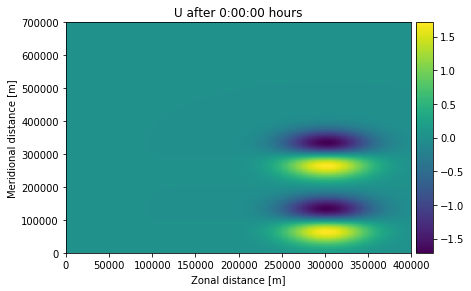The next step is to define a ParticleSet. In this case, we start 2 particles at locations (330km, 100km) and (330km, 280km) using the from_list constructor method, that are advected on the fieldset we defined above. Note that we use JITParticle as pclass, because we will be executing the advection in JIT (Just-In-Time) mode. The alternative is to run in scipy mode, in which case pclass is ScipyParticle

In :
pset = ParticleSet.from_list(fieldset=fieldset,   # the fields on which the particles are advected
pclass=JITParticle,  # the type of particles (JITParticle or ScipyParticle)
lon=[3.3e5,  3.3e5], # a vector of release longitudes
lat=[1e5, 2.8e5])    # a vector of release latitudes


Print the ParticleSet to see where they start

In :
print(pset)

P(lon=330000.000000, lat=100000.000000, depth=0.000000, time=not_yet_set)
P(lon=330000.000000, lat=280000.000000, depth=0.000000, time=not_yet_set)


This output shows for each particle the (longitude, latitude, depth, time). Note that in this case the time is not_yet_set, that is because we didn't specify a time when we defined the pset.

To plot the positions of these particles on the zonal velocity, use the following command

In :
pset.show(field=fieldset.U)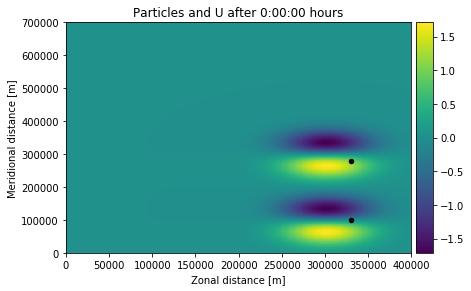The final step is to run (or 'execute') the ParticelSet. We run the particles using the AdvectionRK4 kernel, which is a 4th order Runge-Kutte implementation that comes with Parcels. We run the particles for 6 days (using the timedelta function from datetime), at an RK4 timestep of 5 minutes. We store the trajectory information at an interval of 1 hour in a file called EddyParticles.nc. Because time was not_yet_set, the particles will be advected from the first date available in the fieldset, which is the default behaviour.

In :
output_file = pset.ParticleFile(name="EddyParticles.nc", outputdt=timedelta(hours=1)) # the file name and the time step of the outputs
pset.execute(AdvectionRK4,                 # the kernel (which defines how particles move)
runtime=timedelta(days=6),    # the total length of the run
dt=timedelta(minutes=5),      # the timestep of the kernel
output_file=output_file)

INFO: Compiled JITParticleAdvectionRK4 ==> c:\users\miria\appdata\local\temp\parcels-tmp\2895316a8ee14d0a17a7e4c0e2446f2f.dll


The code should have run, which can be confirmed by printing and plotting the ParticleSet again

In :
print(pset)
pset.show(field=fieldset.U)

P(lon=227199.296875, lat=82140.656250, depth=0.000000, time=518400.000000)
P(lon=261315.203125, lat=320586.781250, depth=0.000000, time=518400.000000)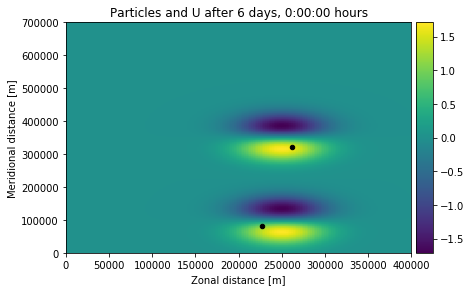Note that both the particles (the black dots) and the U field have moved in the plot above. Also, the time of the particles is now 518400 seconds, which is 6 days.

The trajectory information of the particles can be written to the EddyParticles.nc file by using the .export() method on the output file. The trajectory can then be quickly plotted using the plotTrajectoriesFile function.

In :
output_file.export()
plotTrajectoriesFile('EddyParticles.nc');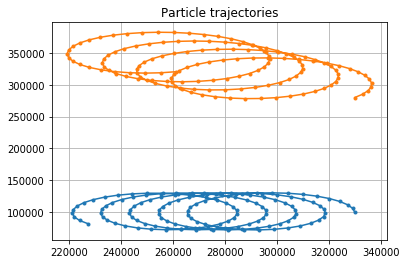The plotTrajectoriesFile function can also be used to show the trajectories as an animation, by specifying that it has to run in movie2d_notebook mode. If we pass this to our function above, we can watch the particles go!

In :
plotTrajectoriesFile('EddyParticles.nc', mode='movie2d_notebook')

Out:

The plotTrajectoriesFile can also be used to display 2-dimensional histograms (mode=hist2d) of the number of particle observations per bin. Use the bins argument to control the number of bins in the longitude and latitude direction. See also the matplotlib.hist2d page.

In :
plotTrajectoriesFile('EddyParticles.nc', mode='hist2d', bins=[30, 20]);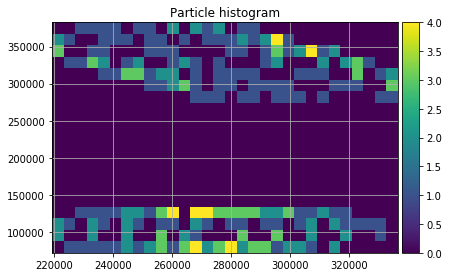Now one of the neat features of Parcels is that the particles can be plotted as a movie during execution, which is great for debugging. To rerun the particles while plotting them on top of the zonal velocity field (fieldset.U), first reinitialise the ParticleSet and then re-execute. However, now rather than saving the output to a file, display a movie using the moviedt display frequency, in this case with the zonal velocity fieldset.U as background

In :
# THIS DOES NOT WORK IN THIS IPYTHON NOTEBOOK, BECAUSE OF THE INLINE PLOTTING.
# THE 'SHOW_MOVIE' KEYWORD WILL WORK ON MOST MACHINES, THOUGH
# pset = ParticleSet(fieldset=fieldset, pclass=JITParticle, lon=[3.3e5, 3.3e5], lat=[1e5, 2.8e5])
#              runtime=timedelta(days=6),
#              dt=timedelta(minutes=5),
#              moviedt=timedelta(hours=1),
#              movie_background_field=fieldset.U)


## Running particles in backward time¶

Running particles in backward time is extremely simple: just provide a dt < 0.

In :
output_file = pset.ParticleFile(name="EddyParticles_Bwd.nc", outputdt=timedelta(hours=1))  # the file name and the time step of the outputs
dt=-timedelta(minutes=5),      # negative timestep for backward run
runtime=timedelta(days=6),     # the run time
output_file=output_file)


Now print the particles again, and see that they (except for some round-off errors) returned to their original position

In :
print(pset)
pset.show(field=fieldset.U)

P(lon=330000.500000, lat=100003.554688, depth=0.000000, time=0.000000)
P(lon=329996.343750, lat=279996.281250, depth=0.000000, time=0.000000)## Adding a custom behaviour kernel¶

A key feature of Parcels is the ability to quickly create very simple kernels, and add them to the execution. Kernels are little snippets of code that are run during exection of the particles.

In this example, we'll create a simple kernel where particles obtain an extra 2 m/s westward velocity after 1 day. Of course, this is not very realistic scenario, but it nicely illustrates the power of custom kernels.

In :
def WestVel(particle, fieldset, time):
if time > 86400:
uvel = -2.
particle.lon += uvel * particle.dt


Now reset the ParticleSet again, and re-execute. Note that we have now changed kernel to be AdvectionRK4 + k_WestVel, where k_WestVel is the WestVel function as defined above cast into a Kernel object (via the pset.Kernel call).

In :
pset = ParticleSet.from_list(fieldset=fieldset, pclass=JITParticle, lon=[3.3e5, 3.3e5], lat=[1e5, 2.8e5])

k_WestVel = pset.Kernel(WestVel)        # casting the WestVel function to a kernel object

output_file = pset.ParticleFile(name="EddyParticles_WestVel.nc", outputdt=timedelta(hours=1))
runtime=timedelta(days=2),
dt=timedelta(minutes=5),
output_file=output_file)

INFO: Compiled JITParticleAdvectionRK4WestVel ==> c:\users\miria\appdata\local\temp\parcels-tmp\88e3bb6347702f6fc0ee477bd063475e.dll


And now plot this new trajectory file

In :
output_file.export()
plotTrajectoriesFile('EddyParticles_WestVel.nc');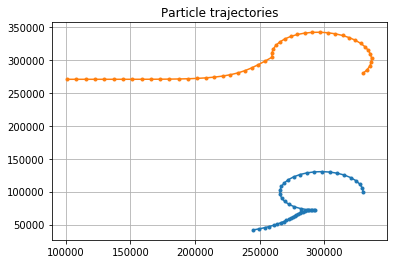## Reading in data from arbritrary NetCDF files¶

In most cases, you will want to advect particles within pre-computed velocity fields. If these velocity fields are stored in NetCDF format, it is fairly easy to load them into the FieldSet.from_netcdf() function.

The examples directory contains a set of GlobCurrent files of the region around South Africa.

First, define the names of the files containing the zonal (U) and meridional (V) velocities. You can use wildcards (*) and the filenames for U and V can be the same (as in this case)

In :
filenames = {'U': "GlobCurrent_example_data/20*.nc",
'V': "GlobCurrent_example_data/20*.nc"}


Then, define a dictionary of the variables (U and V) and dimensions (lon, lat and time; note that in this case there is no depth because the GlobCurrent data is only for the surface of the ocean)

In :
variables = {'U': 'eastward_eulerian_current_velocity',
'V': 'northward_eulerian_current_velocity'}
dimensions = {'lat': 'lat',
'lon': 'lon',
'time': 'time'}


Finally, read in the fieldset using the FieldSet.from_netcdf function with the above-defined filenames, variables and dimensions

In :
fieldset = FieldSet.from_netcdf(filenames, variables, dimensions)

WARNING: Casting lon data to np.float32
WARNING: Casting lat data to np.float32
WARNING: Casting depth data to np.float32


Now define a ParticleSet, in this case with 5 particle starting on a line between (28E, 33S) and (30E, 33S) using the ParticleSet.from_line constructor method

In :
pset = ParticleSet.from_line(fieldset=fieldset, pclass=JITParticle,
size=5,            # releasing 5 particles
start=(28, -33),   # releasing on a line: the start longitude and latitude
finish=(30, -33))  # releasing on a line: the end longitude and latitude


And finally execute the ParticleSet for 10 days using 4th order Runge-Kutta

In :
output_file = pset.ParticleFile(name="GlobCurrentParticles.nc", outputdt=timedelta(hours=6))
runtime=timedelta(days=10),
dt=timedelta(minutes=5),
output_file=output_file)

INFO: Compiled JITParticleAdvectionRK4 ==> c:\users\miria\appdata\local\temp\parcels-tmp\75f2caa4979626927d98dc8a36303c4f.dll


Now visualise this simulation using the plotParticles script again. Note you can plot the particles on top of one of the velocity fields using the tracerfile, tracerfield, etc keywords.

In :
output_file.export()
plotTrajectoriesFile('GlobCurrentParticles.nc',
tracerfile='GlobCurrent_example_data/20020101000000-GLOBCURRENT-L4-CUReul_hs-ALT_SUM-v02.0-fv01.0.nc',
tracerlon='lon',
tracerlat='lat',
tracerfield='eastward_eulerian_current_velocity');

WARNING: Casting time data to np.float64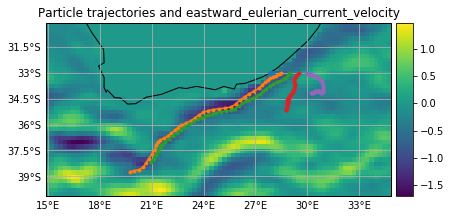## Sampling a Field with Particles¶

One typical use case of particle simulations is to sample a Field (such as temperature, vorticity or sea surface hight) along a particle trajectory. In Parcels, this is very easy to do, with a custom Kernel.

Let's read in another example, the flow around a Peninsula (see Fig 2.2.3 in this document), and this time also load the Pressure (P) field, using extra_fields={'P': 'P'}. Note that, because this flow does not depend on time, we need to set allow_time_extrapolation=True when reading in the fieldset.

In :
fieldset = FieldSet.from_parcels("Peninsula_data/peninsula", extra_fields={'P': 'P'}, allow_time_extrapolation=True)


Now define a new Particle class that has an extra Variable: the pressure. We initialise this by sampling the fieldset.P field.

In :
class SampleParticle(JITParticle):         # Define a new particle class
p = Variable('p', initial=fieldset.P)  # Variable 'p' initialised by sampling the pressure


Now define a ParticleSet using the from_line method also used above in the GlobCurrent data. Plot the pset and print their pressure values p

In :
pset = ParticleSet.from_line(fieldset=fieldset, pclass=SampleParticle,
start=(3000, 3000), finish=(3000, 46000), size=5, time=0)
pset.show(field='vector')
print('p values before execution:', [p.p for p in pset])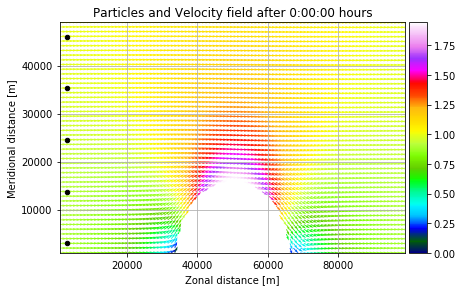('p values before execution:', [-2.6537523, -12.28214, -22.267359, -32.635548, -43.27725])


Now create a custom function that samples the fieldset.P field at the particle location. Cast this function to a Kernel.

In :
def SampleP(particle, fieldset, time):  # Custom function that samples fieldset.P at particle location
particle.p = fieldset.P[time, particle.depth, particle.lat, particle.lon]

k_sample = pset.Kernel(SampleP)    # Casting the SampleP function to a kernel.


Finally, execute the pset with a combination of the AdvectionRK4 and SampleP kernels, plot the pset and print their new pressure values p

In :
pset.execute(AdvectionRK4 + k_sample,    # Add kernels using the + operator.
runtime=timedelta(hours=20),
dt=timedelta(minutes=5))
pset.show(field=fieldset.P, show_time=0)
print('p values after execution:', [p.p for p in pset])

INFO: Compiled SampleParticleAdvectionRK4SampleP ==> c:\users\miria\appdata\local\temp\parcels-tmp\987dbc12bf9604c514878c9ea37cf306.dll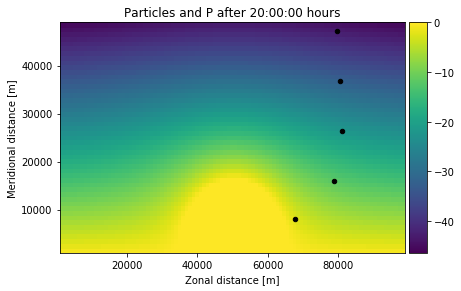('p values after execution:', [-2.65027, -12.282154, -22.267172, -32.635548, -43.277206])


And see that these pressure values p are (within roundoff errors) the same as the pressure values before the execution of the kernels. The particles thus stay on isobars!

Note that there is a crucial subtlety in how to sample time-evolving Fields. In that case, it is important to sample at fieldset.P[time+particle.dt, ...] and to first advect the particles before sampling them. See also this part of the interpolation tutorial for more background.

## Calculating distance travelled¶

As a second example of what custom kernels can do, we will now show how to create a kernel that logs the total distance that particles have travelled.

First, we need to create a new Particle class that includes three extra variables. The distance variable will be written to output, but the auxiliary variables prev_lon and prev_lat won't be written to output (can be controlled using the to_write keyword)

In :
class DistParticle(JITParticle):  # Define a new particle class that contains three extra variables
distance = Variable('distance', initial=0., dtype=np.float32)  # the distance travelled
prev_lon = Variable('prev_lon', dtype=np.float32, to_write=False,
initial=attrgetter('lon'))  # the previous longitude
prev_lat = Variable('prev_lat', dtype=np.float32, to_write=False,
initial=attrgetter('lat'))  # the previous latitude.


Now define a new function TotalDistance that calculates the sum of Euclidean distances between the old and new locations in each RK4 step

In :
def TotalDistance(particle, fieldset, time):
# Calculate the distance in latitudinal direction (using 1.11e2 kilometer per degree latitude)
lat_dist = (particle.lat - particle.prev_lat) * 1.11e2
# Calculate the distance in longitudinal direction, using cosine(latitude) - spherical earth
lon_dist = (particle.lon - particle.prev_lon) * 1.11e2 * math.cos(particle.lat * math.pi / 180)
# Calculate the total Euclidean distance travelled by the particle
particle.distance += math.sqrt(math.pow(lon_dist, 2) + math.pow(lat_dist, 2))

particle.prev_lon = particle.lon  # Set the stored values for next iteration.
particle.prev_lat = particle.lat


Note: here it is assumed that the latitude and longitude are measured in degrees North and East, respectively. However, some datasets (e.g. the MovingEddies used above) give them measured in (kilo)meters, in which case we must not include the factor 1.11e2.

We will run the TotalDistance function on a ParticleSet containing the five particles within the GlobCurrent fieldset from above. Note that pclass=DistParticle in this case.

In :
filenames = {'U': "GlobCurrent_example_data/20*.nc",
'V': "GlobCurrent_example_data/20*.nc"}
variables = {'U': 'eastward_eulerian_current_velocity',
'V': 'northward_eulerian_current_velocity'}
dimensions = {'lat': 'lat',
'lon': 'lon',
'time': 'time'}
fieldset = FieldSet.from_netcdf(filenames, variables, dimensions)
pset = ParticleSet.from_line(fieldset=fieldset,
pclass=DistParticle,
size=5, start=(28, -33), finish=(30, -33))


Again define a new kernel to include the function written above and execute the ParticleSet.

In :
k_dist = pset.Kernel(TotalDistance)  # Casting the TotalDistance function to a kernel.

runtime=timedelta(days=6),
dt=timedelta(minutes=5),
output_file=pset.ParticleFile(name="GlobCurrentParticles_Dist.nc", outputdt=timedelta(hours=1)))

INFO: Compiled DistParticleAdvectionRK4TotalDistance ==> c:\users\miria\appdata\local\temp\parcels-tmp\1bd8ef2e6f3ce7fb4fc5f2ff255b6b6a.dll


And finally print the distance in km that each particle has travelled (note that this is also stored in the EddyParticles_Dist.nc file)

In :
print([p.distance for p in pset]) #the distances in km travelled by the particles

[13.197482, 641.25183, 543.7125, 183.66846, 172.78123]